# Introduction

As stated in the introduction to this package, one of the key contributions of voluModel is the way it handles the extraction of environmental data for niche modeling. Specifically, environmental data at presences, absences, pseudoabsences, and/or background points can be extracted from environmental data RasterBrick objects based on depth in addition to horizontal coordinates. Another useful tool provided with voluModel is marineBackground(), which helps the user generate training regions from which background or pseudoabsence data are drawn. This is where we will start, so let’s get into it.

Here are the packages you will need.

library(voluModel) # Because of course
library(ggplot2) # For fancy plotting
library(rgdal,
options("rgdal_show_exportToProj4_warnings"="none")) # For vector stuff. Will eventually be replaced with sf.
library(raster) # For raster stuff. Will eventually be replaced with terra.
library(rangeBuilder) # To compare marineBackground to getDynamicAlphaHull
library(dplyr) # To filter data

# Generating Repeatable Training Regions

To generate a sample of pseudoabsences and/or background points, it is important to consider the environments the species of interest can access (Barve et al., 2011). There is ample discussion in the literature on how to best delimit a niche model training region (also variously called M or the background region), and we will not review the issue here. In the absence of specific information on species’ dispersal capabilities, algorithmically generating a repeatable sampling background from a clear set of rules may be suitable approximation, or a jumping-off point for hand-curating training regions in a GIS software of choice.

We designed marineBackground() as a wrapper around , a function provided by the rangeBuilder package (Davis-Rabosky et al., 2016) which generates background sampling regions by fitting an alpha hull polygon around an occurrence point dataset. Let’s look at how marineBackground() (and by extension getDynamicAlphaHull()) fits alpha hull polygons, based on species occurrence data. The occurrence dataset is our Steindachneria argentea, Luminous Hake, data downloaded via R (R Core Team, 2020) from GBIF (Chamberlain et al., 2021; Chamberlain and Boettiger, 2017) and OBIS (Provoost and Bosch, 2019) via occCite (Owens et al., 2021).

# Get points
package='voluModel'))
occurrences <- occs %>%
dplyr::select(decimalLongitude, decimalLatitude, depth) %>%
dplyr::distinct() %>%
dplyr::filter(dplyr::between(depth, 1, 2000))

# Map occurrences
land <- rnaturalearth::ne_countries(scale = "small", returnclass = "sf")
pointMap(occs = occurrences, ptCol = "orange", landCol = "black",
spName = "Steindachneria argentea", ptSize = 3,
land = land)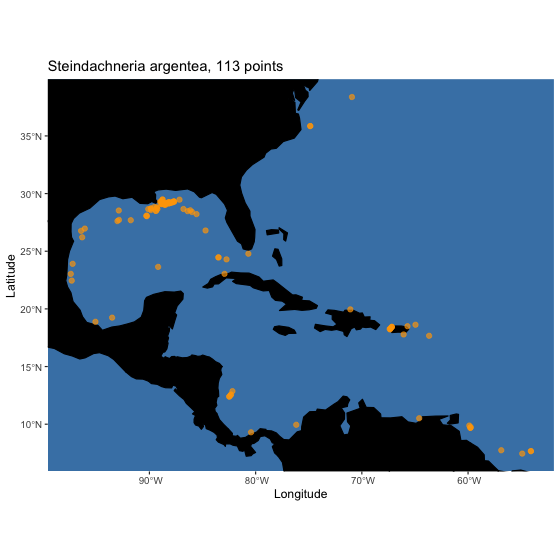getDynamicAlphaHull(), which is run within marineBackground() generates a polygon (or polygons) that can be used as a training region by fitting an alpha hull (or hulls) around an occurrence dataset. Alpha is a parameter that controls the complexity of the polygon(s) that is being fit around the occurrences. getDynamicAlphaHull() adjusts the alpha parameter iteratively, until it meets criteria the user can set via the arguments fraction, which is the minimum fraction of occurrences that must be contained within the polygon(s), partCount, which is the maximum number of polygons generated to fit the data, and buff, which is the permissible distance from a point to the edge of a polyon in meters. marineBackground() takes these and many of the same other arguments as getDynamicAlphaHull(). Here’s how it works.

trainingRegion <- marineBackground(occurrences,
fraction = 1, partCount = 1, buff = 1000000,
clipToOcean = F)
plot(occurrences[,c("decimalLongitude", "decimalLatitude")],
main = "Mimimum of 100% Points in Training Region,\nMaximum of 1 Polygon Permitted, 100 km Buffer",
xlab = "Longitude", ylab = "Latitude",
pch = 20, col = "red",
xlim = c(-105, -40), ylim = c(0, 45))
plot(land, col = "black", add = T)
plot(trainingRegion, add = T, border = "orange", lwd = 2)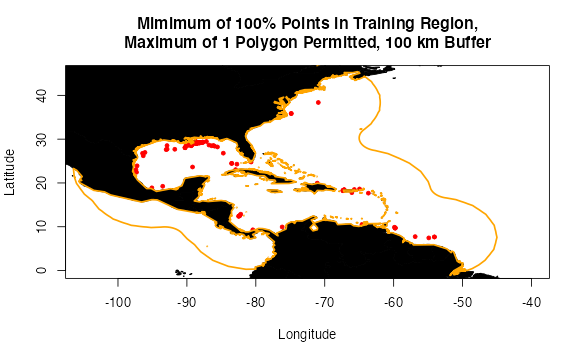What parameters you choose to fit the alpha hull will depend on the biology of the organism you are trying to model (e.g. you may choose a much smaller buffer for a sessile organism than you would for a highly vagile migratory species), your data, and the particulars of your study system and the geography of your study area. You may need to experiment to find something that looks sensible. If you do not provide a pre-defined buffer, marineBackground() will calculate a buffer that is the mean of the top and bottom 10% of distances between points in the dataset.

You may have noticed that the training regions in the above examples include some areas of the Pacific where the species has not been observed, and cannot access. This could cause problems if it is used to train your model. You could delete this area by hand in your favorite GIS software, OR you could change clipToOcean to TRUE in marineBackground(), and any polygon fragments that do not contain an occurrence will be deleted automatically. This is a key difference from getDynamicAlphaHull().

trainingRegion <- marineBackground(occurrences,
buff = 1000000,
clipToOcean = T)
plot(occurrences[,c("decimalLongitude", "decimalLatitude")],
main = "100 km Buffer,\n Training Region Clipped to Occupied Polygon",
xlab = "Longitude", ylab = "Latitude",
pch = 20, col = "red",
xlim = c(-105, -40), ylim = c(0, 45))
plot(land, col = "black", add = T)
plot(trainingRegion, add = T, border = "orange", lwd = 2)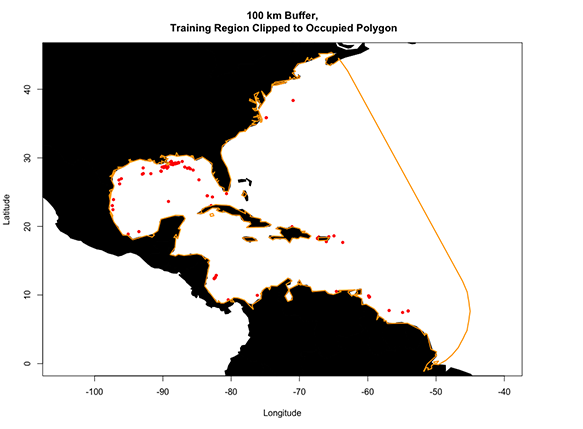The last major difference between marineBackground() and getDynamicAlphaHull() is how the functions handle training regions that are adjacent to the antimeridian (i.e. 180° E or W). getDynamicAlphaHull() truncates polygons at this line, which can lead to some strange-looking training areas or fatal errors. marineBackground(), on the other hand, wraps polygons across the meridian and merges them if they overlap. For this example, I am going to manipulate the longitudinal coordinates of our Luminous Hake dataset to generate a quick fictional example.

# Fictional example occurrences
pacificOccs <- occurrences
pacificOccs$decimalLongitude <- pacificOccs$decimalLongitude - 100
for (i in 1:length(pacificOccs$decimalLongitude)){ if (pacificOccs$decimalLongitude[[i]] < -180){
pacificOccs$decimalLongitude[[i]] <- pacificOccs$decimalLongitude[[i]] + 360
}
}

# marine Background
pacificTrainingRegion <- marineBackground(pacificOccs,
fraction = 1, partCount = 2,
clipToOcean = T)
plot(pacificOccs[,c("decimalLongitude", "decimalLatitude")],
main = "marineBackground Antimeridian Wrap",
xlab = "Longitude", ylab = "Latitude",
ylim = c(-40,60),
pch = 20, col = "red")
plot(land, col = "black", add = T)
plot(pacificTrainingRegion, add = T, border = "orange", lwd = 2)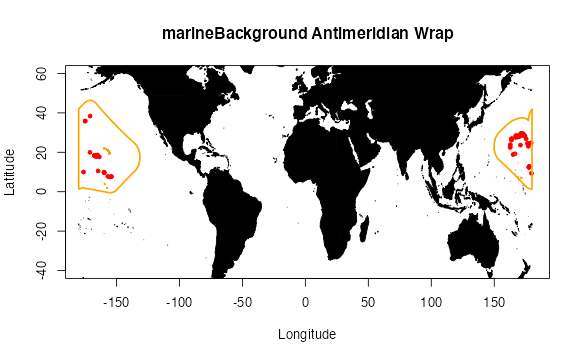# 3D Data Extraction

Next, we will cover how to extract environmental data from a RasterBrick object using 3D occurrence coordinates. The occurrences I will use to demonstrate this are the Luminous Hake occurrences from the previous section. The environmental dataset I will use as an example is temperature (Locarnini et al., 2018) from the World Ocean Atlas (WOA; Garcia et al., 2019). I have chosen to focus on temperature for simple illustrative purposes–we recommend you explore additional variables from the World Ocean Atlas and other sources. These data are supplied by the World Ocean Atlas as point shapefiles; the version supplied here has been cropped between -110 and -40 longitude and between -5 and 50 latitude to make it more memory-efficient.

This chunk of code loads the example shapefile, which results in a SpatialPointsDataFrame:

td <- tempdir()
unzip(system.file("extdata/woa18_decav_t00mn01_cropped.zip",
package = "voluModel"),
exdir = paste0(td, "/temperature"), junkpaths = T)
temperature <- readOGR(dsn = paste0(td, "/temperature"),
layer ="woa18_decav_t00mn01_cropped")
## OGR data source with driver: ESRI Shapefile
## Source: "/private/var/folders/bn/2vhstsbj041cx84rt_n8rv080000gp/T/RtmpX8ubzQ/temperature", layer: "woa18_decav_t00mn01_cropped"
## with 1633 features
## It has 102 fields
unlink(paste0(td, "/temperature"), recursive = T)

temperature@data[temperature@data == -999.999] <- NA

# How are the data structured?
head(temperature@data)
##   SURFACE    d5M   d10M   d15M   d20M   d25M   d30M   d35M   d40M   d45M   d50M
## 1  23.514 23.266 22.969 22.373 21.832 21.320 20.839 20.309 19.780 19.264 18.715
## 2  23.542 23.222 22.915 22.430 21.938 21.343 20.747 20.182 19.584 18.984 18.394
## 3  23.575 23.392 22.919 22.318 21.766 21.117 20.531 19.860 19.266 18.673 18.161
## 4  23.204 22.979 22.559 22.057 21.401 20.651 19.978 19.433 18.835 18.247 17.691
## 5  23.451 23.125 22.726 22.134 21.520 20.828 20.128 19.472 18.875 18.353 17.875
## 6  23.261 22.968 22.559 22.052 21.528 20.945 20.337 19.759 19.198 18.669 18.162
##     d55M   d60M   d65M   d70M   d75M   d80M   d85M   d90M   d95M  d100M  d125M
## 1 18.164 17.671 17.179 16.743 16.284 15.861 15.622 15.217 14.949 14.767 14.020
## 2 17.843 17.343 16.906 16.497 16.113 15.800 15.488 15.187 14.927 14.720 13.958
## 3 17.675 17.174 16.689 16.236 15.919 15.619 15.391 15.165 14.931 14.699 13.993
## 4 17.177 16.744 16.395 16.074 15.633 15.361 15.172 14.891 14.735 14.556 13.907
## 5 17.425 16.966 16.536 16.184 15.881 15.588 15.335 15.110 14.875 14.686 14.084
## 6 17.727 17.306 16.856 16.491 16.135 15.794 15.530 15.219 15.031 14.822 14.159
##    d150M  d175M  d200M  d225M  d250M  d275M  d300M  d325M  d350M  d375M d400M
## 1 13.552 13.277 13.096 12.912 12.678 12.306 11.736 11.055 10.482  9.987 9.504
## 2 13.598 13.302 13.106 12.928 12.667 12.272 11.675 11.060 10.469  9.908 9.476
## 3 13.574 13.328 13.123 12.928 12.693 12.278 11.729 11.155 10.538  9.984 9.504
## 4 13.528 13.305 13.117 12.926 12.655 12.275 11.745 11.064 10.454  9.927 9.559
## 5 13.720 13.465 13.216 13.032 12.682 12.247 11.732 11.089 10.423  9.892 9.668
## 6 13.757 13.515 13.299 13.123 12.759 12.287 11.760 11.087 10.540 10.019 9.684
##   d425M d450M d475M d500M d550M d600M d650M d700M d750M d800M d850M d900M d950M
## 1 9.061 8.696 8.413 8.119 7.570 7.117 6.676 6.241 5.894 5.618 5.313 5.062 4.828
## 2 9.071 8.712 8.431 8.134 7.621 7.136 6.644 6.255 5.891 5.574 5.262 4.994 4.787
## 3 9.093 8.765 8.442 8.101 7.620 7.180 6.687 6.267 5.875 5.566 5.293 5.052 4.811
## 4 9.118 8.732 8.421 8.182 7.663 7.176 6.712 6.270 5.912 5.598 5.330 5.094 4.844
## 5 9.063 8.720 8.404 8.156 7.593 7.114 6.662 6.249 5.869 5.553 5.301 5.038 4.781
## 6 9.142 8.805 8.477 8.217 7.654 7.170 6.760 6.335 5.927 5.590 5.327 5.088 4.847
##   d1000M d1050M d1100M d1150M d1200M d1250M d1300M d1350M d1400M d1450M d1500M
## 1  4.618  4.410  4.223  4.054  3.868  3.727  3.563  3.410  3.278  3.144  3.050
## 2  4.562  4.379  4.207  4.048  3.876  3.731  3.560  3.428  3.297  3.167  3.040
## 3  4.571  4.397  4.218  4.048  3.896  3.737  3.592  3.440  3.286  3.149  3.044
## 4  4.614  4.415  4.219  4.048  3.899  3.743  3.581  3.426  3.279  3.153  3.049
## 5  4.587  4.395  4.233  4.062  3.911  3.727  3.555  3.404  3.279  3.150  3.045
## 6  4.617  4.441  4.243  4.060  3.905  3.747  3.573  3.424  3.294  3.172  3.050
##   d1550M d1600M d1650M d1700M d1750M d1800M d1850M d1900M d1950M d2000M d2100M
## 1  2.949  2.858  2.769  2.692  2.618  2.540  2.470  2.406  2.329  2.320  2.227
## 2  2.950  2.856  2.765  2.686  2.606  2.527  2.455  2.376  2.308     NA     NA
## 3  2.946  2.860  2.759  2.682  2.605  2.531  2.456  2.392  2.326  2.351  2.193
## 4  2.938  2.843  2.754  2.675  2.602  2.528  2.463  2.398  2.335  2.223  2.130
## 5  2.936  2.845  2.760  2.666  2.579  2.513  2.453  2.395  2.340  2.254  2.165
## 6  2.946  2.845  2.758  2.679  2.600  2.526  2.459  2.397  2.338  2.276  2.165
##   d2200M d2300M d2400M d2500M d2600M d2700M d2800M d2900M d3000M d3100M d3200M
## 1  2.147  2.075  2.012  1.960  1.908  1.864  1.826  1.794  1.770     NA     NA
## 2     NA     NA     NA     NA     NA     NA     NA     NA     NA     NA     NA
## 3  2.081  1.993  1.921  1.862  1.813  1.777  1.753  1.754  1.755     NA     NA
## 4  2.046  1.962  1.891  1.834  1.789  1.795  1.745     NA     NA     NA     NA
## 5  2.047  1.985  1.921  1.870  1.812  1.769  1.746  1.743  1.753  1.766     NA
## 6  2.076  2.003  1.936  1.886  1.843  1.796  1.761  1.751  1.753  1.759   1.78
##   d3300M d3400M d3500M d3600M d3700M d3800M d3900M d4000M d4100M d4200M d4300M
## 1     NA     NA     NA     NA     NA     NA     NA     NA     NA     NA     NA
## 2     NA     NA     NA     NA     NA     NA     NA     NA     NA     NA     NA
## 3     NA     NA     NA     NA     NA     NA     NA     NA     NA     NA     NA
## 4     NA     NA     NA     NA     NA     NA     NA     NA     NA     NA     NA
## 5     NA     NA     NA     NA     NA     NA     NA     NA     NA     NA     NA
## 6     NA     NA     NA     NA     NA     NA     NA     NA     NA     NA     NA
##   d4400M d4500M d4600M d4700M d4800M d4900M d5000M d5100M d5200M d5300M d5400M
## 1     NA     NA     NA     NA     NA     NA     NA     NA     NA     NA     NA
## 2     NA     NA     NA     NA     NA     NA     NA     NA     NA     NA     NA
## 3     NA     NA     NA     NA     NA     NA     NA     NA     NA     NA     NA
## 4     NA     NA     NA     NA     NA     NA     NA     NA     NA     NA     NA
## 5     NA     NA     NA     NA     NA     NA     NA     NA     NA     NA     NA
## 6     NA     NA     NA     NA     NA     NA     NA     NA     NA     NA     NA
##   d5500M
## 1     NA
## 2     NA
## 3     NA
## 4     NA
## 5     NA
## 6     NA

As you can see, each point is a line in the SpatialPointsDataFrame; each column is a depth layer at that point–“d5M” translates to “5 meters deep”. For more details on how to process environmental data, see the raster data tutorial. This next chunk converts the SpatialPointsDataFrame to a RasterBrick; the layers of the RasterBrick are then named the same as the column names of the SpatialPointsDataFrame. I strip the “d” and “M” from the column names so they are a little easier to use as depth coordinates. Note that R will not allow the raster names to be numeric, so it adds an “X” to the beginning of each column name. Don’t worry about this–voluModel can handle numbers preceded by “X”s without user intervention.

# Creating a RasterBrick
temperature <- rasterFromXYZ(cbind(temperature@coords,
temperature@data))

# Get names of depths
envtNames <- gsub("[d,M]", "", names(temperature))
envtNames[] <- "0"
names(temperature) <- envtNames

# Here's a sampling of depth plots from the 102 depth layers available
plot(temperature[[c(1, 50)]])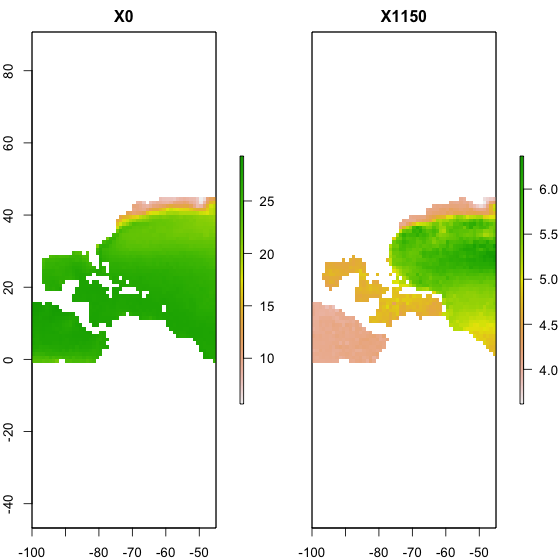At this point, you may have noticed a message popping up about which columns voluModel is interpreting as coordinates. voluModel is fairly flexible in how the coordinate columns are named, and it relies on names instead of column position to interpret x, y, and z (or latitude, longitude, and depth) coordinates. We do this to make voluModel as flexible as possible to a diversity of input formats, although English is the only supported language at the moment. Here are some examples of names that work.

occsTest <- occurrences[1:5,]
xyzSample(occs = occsTest, envBrick = temperature)
## Using decimalLongitude, decimalLatitude, and depth
##  as x, y, and z coordinates, respectively.
##  NA NA NA NA NA
colnames(occsTest) <- c("x", "y", "z")
xyzSample(occs = occsTest, envBrick = temperature)
## Using x, y, and z
##  as x, y, and z coordinates, respectively.
##  NA NA NA NA NA
rm(occsTest)

One optional step in a niche modeling workflow is to down-sample occurrence data to the same resolution as the environmental data. We do this to avoid over-fitting the model due to biased sampling. The next chunk of code downsamples occurrence points so that there is only one per voxel (i.e. 3D pixel) of environmental data. It does this by looping through each layer of the RasterBrick, selecting the occurrence points with depths closest to the depths represented by that layer in the raster brick. I then downsample these points using voluModel’s downsample() function.

# Gets the layer index for each occurrence by matching to depth
layerNames <- as.numeric(gsub("[X]", "", names(temperature)))
occurrences$index <- unlist(lapply(occurrences$depth, FUN = function(x) which.min(abs(layerNames - x))))
indices <- unique(occurrences$index) downsampledOccs <- data.frame() for(i in indices){ tempPoints <- occurrences[occurrences$index==i,]
tempPoints <- downsample(tempPoints, temperature[])
tempPoints$depth <- rep(layerNames[[i]], times = nrow(tempPoints)) downsampledOccs <- rbind(downsampledOccs, tempPoints) } occurrences <- downsampledOccs head(occurrences) ## decimalLongitude decimalLatitude depth ## 1 -54.5 7.5 250 ## 2 -54.5 7.5 450 ## 3 -82.5 12.5 450 ## 4 -93.5 19.5 450 ## 5 -97.5 22.5 450 ## 6 -82.5 23.5 450 print(paste0("Original number of points: ", nrow(occs), "; number of downsampled occs: ", nrow(occurrences))) ##  "Original number of points: 301; number of downsampled occs: 82" The resampled points returned by downsample() are horizontally centered in each voxel. The red dots in the plot show occurrences from the original dataset; the orange dots show the downsampled dataset. Not all of the orange dots may be visible underneath the red dots. pointCompMap(occs1 = occs, occs2 = occurrences, occs1Name = "Original", occs2Name = "Cleaned", spName = "Steindachneria argentea", land = land) ## Using decimalLongitude and decimalLatitude ## as x and y coordinates, respectively. ## Using decimalLongitude and decimalLatitude ## as x and y coordinates, respectively. ## Joining, by = c("decimalLongitude", "decimalLatitude") ## Joining, by = c("decimalLongitude", "decimalLatitude") ## Joining, by = c("decimalLongitude", "decimalLatitude")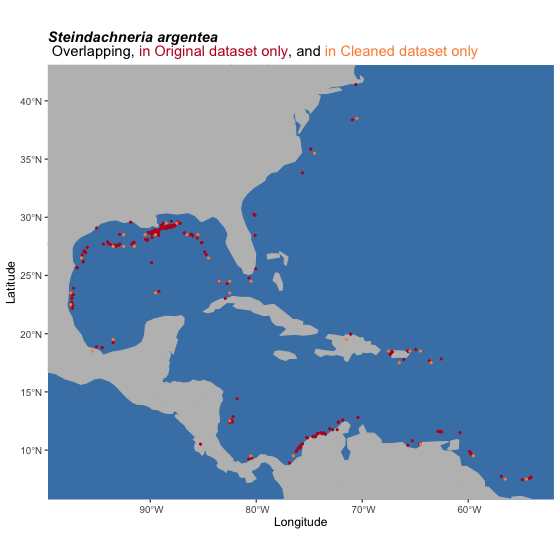At this point, we can demonstrate one of the key contributions of voluModel– an improvement in how the environmental data are extracted at species’ occurrences. voluModel samples environmental conditions at the depth where each record was located, instead of extrapolating to surface or bottom conditions. Finally, we add a column called “response” and fill it by repeating “1”, to signify that these occurrences should be interpreted as presences for the purposes of modeling. # Extraction occurrences$temperature <- xyzSample(occs = occurrences, envBrick = temperature)
## Using decimalLongitude, decimalLatitude, and depth
##  as x, y, and z coordinates, respectively.
# Add "response" column for modeling
occurrences$response <- rep(1, times = nrow(occurrences)) occurrences <- occurrences[complete.cases(occurrences),] head(occurrences) ## decimalLongitude decimalLatitude depth temperature response ## 4 -93.5 19.5 450 9.137 1 ## 6 -82.5 23.5 450 11.395 1 ## 7 -89.5 23.5 450 9.285 1 ## 8 -84.5 26.5 450 9.145 1 ## 10 -91.5 27.5 450 9.091 1 ## 18 -67.5 18.5 375 15.952 1 In a “real” modeling scenario, you would extract all your environmental variables using xyzSample(), then add the response column. Or add the response column first or in the middle. I’m not the boss of you. # Background Data Extraction As discussed above, some modeling methods require the generation of a sampling region from which training/pseudoabsence/background points are drawn. The training region should approximately answer the question “What environments are most likely to have been experienced by Lumious Hakes?” Our answer to this question for the purposes of this tutorial is represented in trainingRegion, which we generated above using marineBackground(). Next, we generate 3D training points within this region using the mSampling3D() function. The function takes an occurrence data.frame, a RasterBrick that will serve as a template for the resolution of the training points, a SpatialPolygons object representing the training region, and, optionally, information on the range of depths from which the function should sample. If you have reason to believe the species you are modeling can access depths higher or lower than those represented by occurrences, you can specify a maximum and minimum training depth from which to draw occurrence points. mSampling3D() by default draws training points from the full depth extent of the supplied RasterBrick. For this example, I am sampling training points from 50 to 1500 m. # Background backgroundVals <- mSampling3D(occs = occurrences, envBrick = temperature, mShp = trainingRegion, depthLimit = c(50, 1500)) ## Using decimalLongitude, decimalLatitude, and depth ## as x, y, and z coordinates, respectively. backgroundVals$temperature <- xyzSample(occs = backgroundVals, temperature)
## Using decimalLongitude, decimalLatitude, and depth
##  as x, y, and z coordinates, respectively.

Once the points are sampled, we add a column called “response” and fill it by repeating “0”, to signify that these occurrences should be interpreted as absences for the purposes of modeling.

# Add "response" column for modeling
backgroundVals$response <- rep(0, times = nrow(backgroundVals)) backgroundVals <- backgroundVals[complete.cases(backgroundVals),] head(backgroundVals) ## decimalLongitude decimalLatitude depth temperature response ## 1 -68.5 43.5 50 6.473 0 ## 2 -67.5 43.5 50 6.669 0 ## 3 -66.5 43.5 50 6.779 0 ## 4 -64.5 43.5 50 3.679 0 ## 5 -63.5 43.5 50 4.003 0 ## 6 -69.5 42.5 50 6.187 0 It is important to note that this background data represents EVERY voxel within the training region that is not occupied by observances. Depending on your study design and the modeling algorithm you are using, these data will need to be subsampled. You could choose a random sample, select points based on geography, or any number of other strategies. Below is just one example–weighting the background points by their environmental dissimilarity to the Luminous Hake occurrence points. This manner of downsampling may be appropriate for modeling methods that interpret training points as pseudoabsences, such as generalized linear modeling, but will lead to overfit models when using algorithms like Maxent. # Sample background points weighted by distance from mean of occurrence temperatures meanTemp <- mean(occurrences[,c("temperature")]) backgroundVals$distance <- abs(backgroundVals[,"temperature"] - meanTemp)
backgroundVals$sampleWeight <- (backgroundVals$distance -
min(backgroundVals$distance))/(max(backgroundVals$distance) -
min(backgroundVals$distance)) sampleForAbsence <- sample(x = rownames(backgroundVals), size = nrow(occurrences) * 100, prob = backgroundVals$backgroundVals)
backgroundVals <- backgroundVals[match(sampleForAbsence,
rownames(backgroundVals)),]

backgroundVals$response <- as.factor(backgroundVals$response)

# Unite datasets and see how things look
dataForModeling <- rbind(occurrences, backgroundVals[,colnames(occurrences)])
ggplot(dataForModeling, aes(x = temperature, fill = response, color = response)) +
geom_density(alpha = .6) +
scale_color_manual(values=c("#999999", "#E69F00", "#56B4E9"),
labels = c("Pseudoabsence", "Presence")) +
scale_fill_manual(values=c("#999999", "#E69F00", "#56B4E9"),
labels = c("Pseudoabsence", "Presence")) +
labs(title="Temperature Sampling Density,\nOccurrences vs. Pseudoabsences",
x="Temperature (C)", y = "Density")+
theme_classic()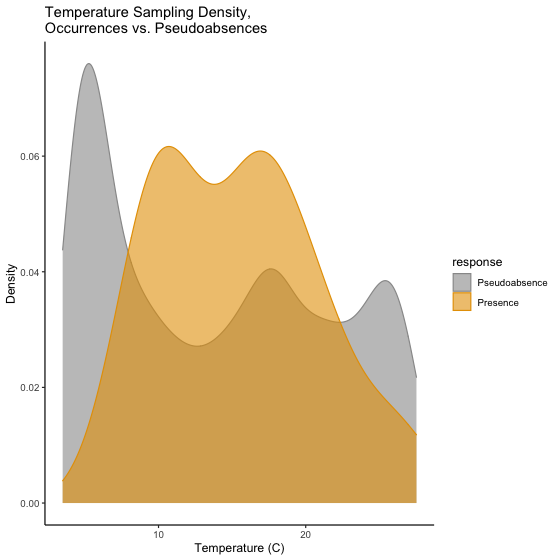For a more complex, multivariate example of environmental dissimilarity weighting, refer to the GLM workflow.